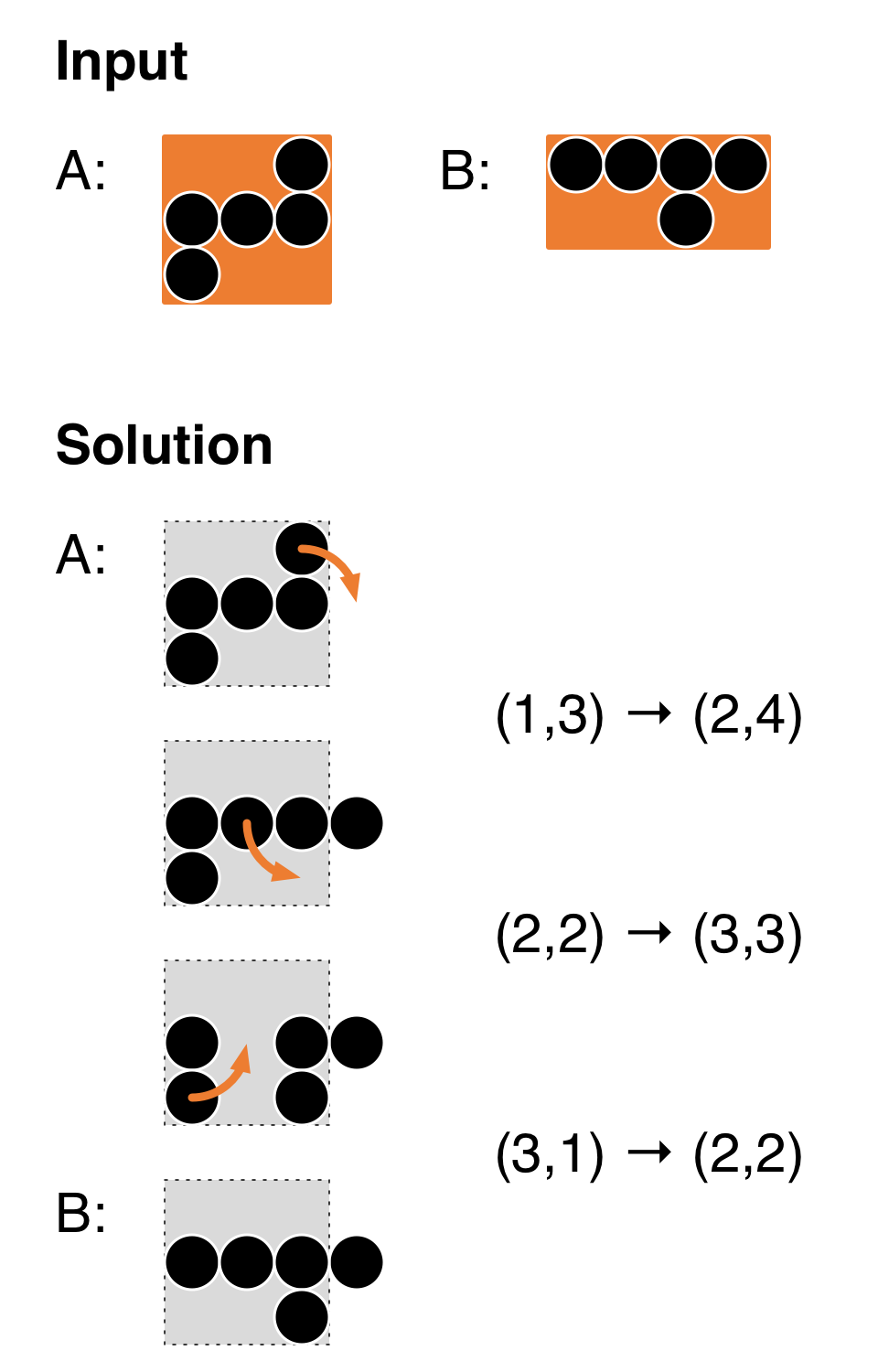Code Submission Evaluation System Login

HIIT Open 2017

 Start: 2017-05-27 11:00:00 End: 2017-05-27 16:00:00

CSES - HIIT Open 2017 - ImpatienceCSES - Impatience

Impatience

 Time limit: 2.00 s Memory limit: 512 MB

Impatience is a one-person game played on an infinitely large 2-dimensional grid. Each grid cell is either empty or it contains one pebble. We refer to grid cells with coordinates $(r,c)$, where $r$ is the row (numbered from top to bottom) and $c$ is the column (numbered from left to right).

You are given two shapes, A and B, that show the positions of the pebbles. In the beginning, you place all pebbles according to shape A, with the top left corner in cell $(1,1)$. Then you can perform a sequence of valid rotations in which you move one pebble to a new position. You win if you find a sequence of rotations so that your final configuration looks like shape B (possibly shifted horizontally and vertically, but not rotated or mirrored).

A valid rotation is defined as follows. Let $r_1$ and $r_2$ be two adjacent rows (i.e., $|r_1-r_2| = 1$) and let $c_1$ and $c_2$ be two adjacent columns (i.e., $|c_1-c_2| = 1$). Now assume that:
• $(r_1,c_1)$ contains a pebble
• $(r_2,c_2)$ is empty
• exactly one of $(r_1,c_2)$ and $(r_2,c_1)$ contains a pebble.
Then we can move a pebble from $(r_1,c_1)$ to $(r_2,c_2)$; such a move is denoted by $(r_1,c_1) \to (r_2,c_2)$. See the figure below for an example of inputs and a sequence of 3 valid rotations that wins the game.Note that you are free to use both negative and positive coordinates. You can freely step outside the boundaries of the images A and B.

Input

As input, you will receive two shapes. Each shape is encoded as follows:
• One line with two numbers $n$ and $m$: the height and the width of the shape.
• $n$ lines, with $m$ characters on each line. The characters are either 0 or 1, indicating an empty slot or a slot with a pebble, respectively.
The shapes do not have any holes (starting from any empty slot, you can reach another empty slot by a sequence of moves up/down/left/right through empty slots).

Output

If there is no way to win the game, output just -1.

Otherwise, output a sequence of valid rotations that wins the game, encoded as follows. First, output one line which contains just one number $k$, the number of rotations. Then, output $k$ lines of the form "$r_1$ $c_1$ $r_2$ $c_2$", which indicates a rotation $(r_1,c_1) \to (r_2,c_2)$.

You can use at most 200000 rotations in your output.

Limits
• $1 \le n \le 15$
• $1 \le m \le 15$
• The number of pebbles in each shape is between 1 and 100.
Example 1

The following example corresponds to the above illustration.

Input:
3 3 001 111 100 2 4 1111 0010

Output:
3 1 3 2 4 2 2 3 3 3 1 2 2

Example 2

Input:
2 5 00000 11000 3 3 011 000 000

Output:
0

Example 3

Input:
3 13 0101010101110 0111010100100 0101010100100 1 1 1

Output:
-1

Example 4

Input:
1 3 111 3 2 01 01 10

Output:
3 1 3 0 2 1 2 0 3 0 3 -1 2